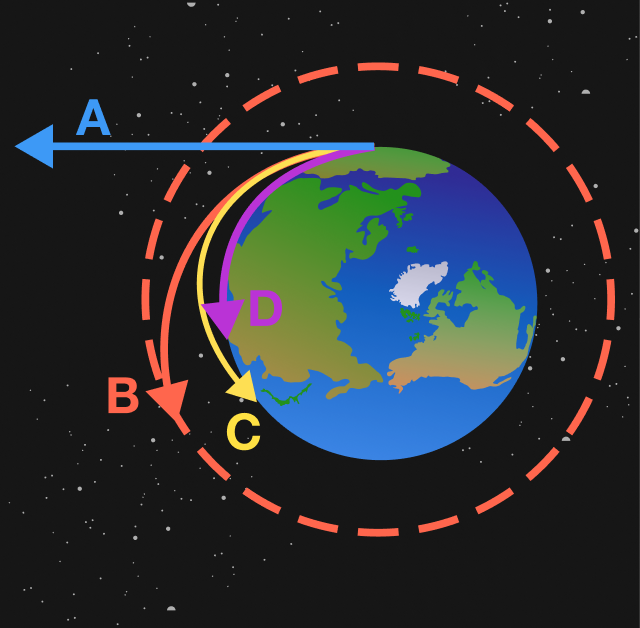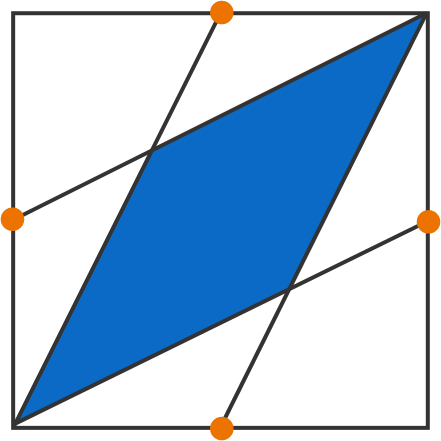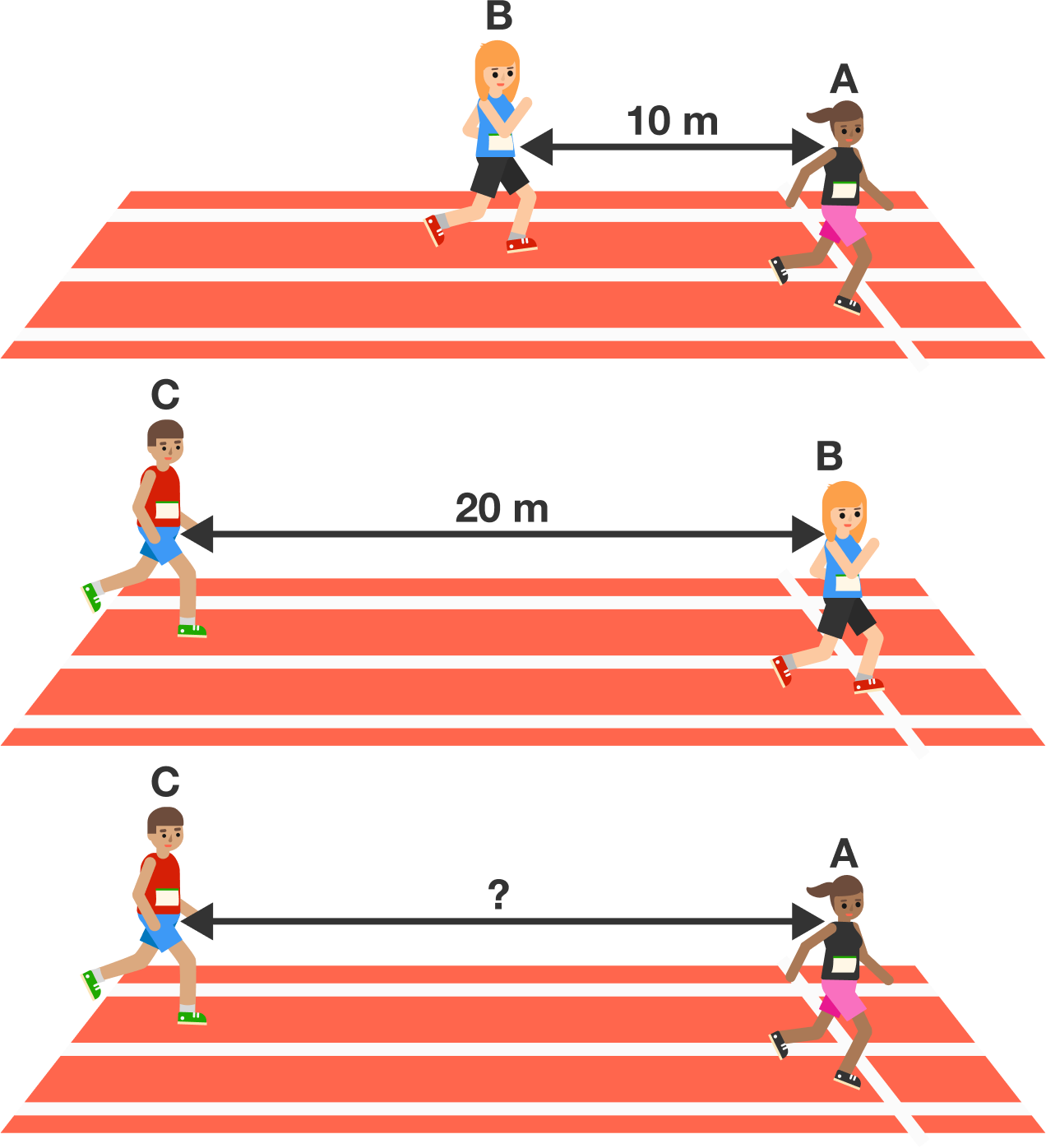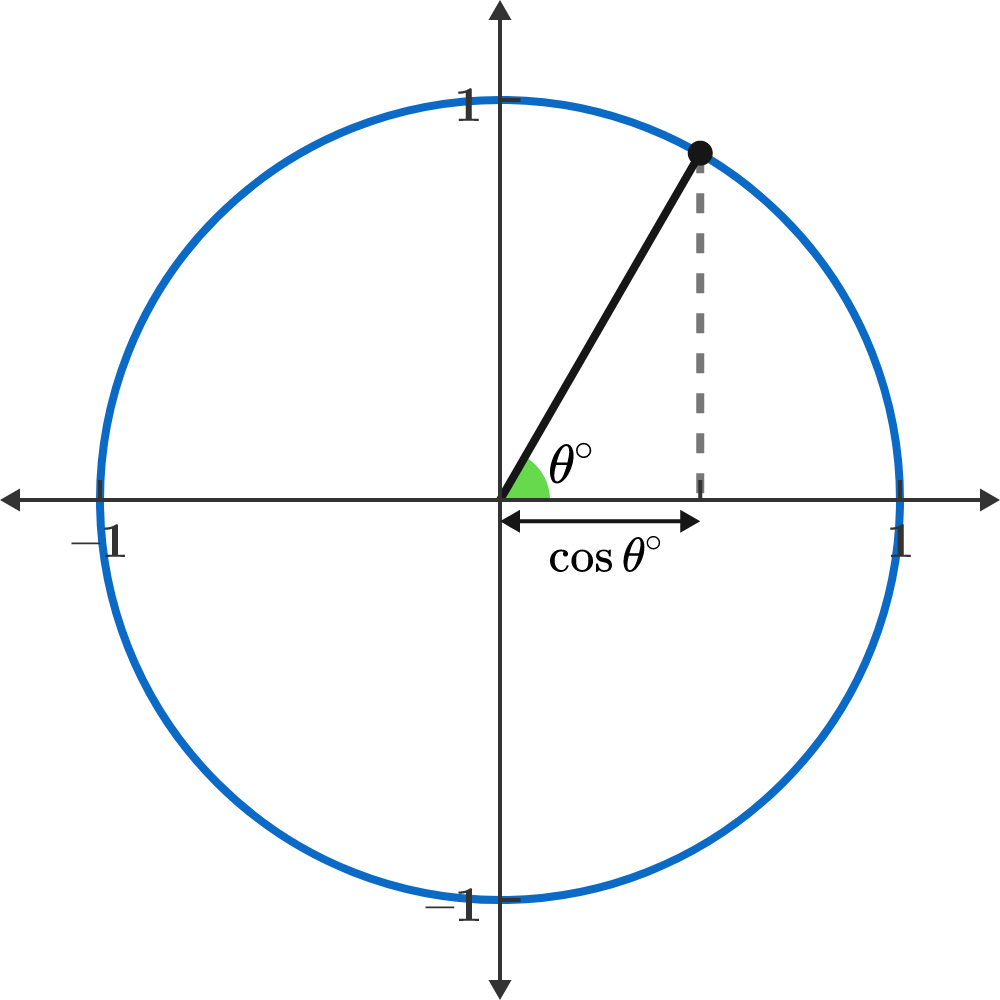# Problems of the Week

Contribute a problem

# 2018-05-14 Basic

If Earth suddenly stopped spinning, mayhem would ensue.

What would happen to the objects on the surface?For simplicity, assume that the objects start near the equator.

$A$ and $B$ are positive real numbers such that $A > B,\quad A^2+B^2=34,\quad AB=15.$ What is $A^2-B^2?$The orange points are the midpoints of the sides of a $6\times 6$ square.

What is the area of the portion shaded blue?

In a race run by A and B, runner A beats B by 10 meters. When runners B and C run the same lap, B beats C by 20 meters.

What happens when runners A and C run the lap?Note: Assume each runner's speed is constant.

$\cos \theta^\circ + \cos (\theta^\circ+1^\circ) + \cos (\theta^\circ+2^\circ) +\cdots + \cos (\theta^\circ+359^\circ)$

What is the value of this expression when $\theta$ is an integer?A certain theta value is shown here, but theta can be any integer. Consider how the cosine changes as the angle increases.

×